One of these questions will ask you about the ratio of books in a library.

# Ratio, Proportion and Percentages

This Math quiz is called 'Ratio, Proportion and Percentages' and it has been written by teachers to help you if you are studying the subject at middle school. Playing educational quizzes is a fabulous way to learn if you are in the 6th, 7th or 8th grade - aged 11 to 14.

It costs only \$12.50 per month to play this quiz and over 3,500 others that help you with your school work. You can subscribe on the page at Join Us

Ratios are used to compare quantities of 2 or more things. Although you are most likely to see them written using the ‘colon’ format, such as 1:3, you should also be able to work with them as fractions. Proportion is used to compare two ratios. Percentages pop up everywhere, and you should be comfortable working with them – don’t forget, they are just a special fraction where everything is out of 100! See how well you know these three topics in this middle school Math quiz.

Ratios can be used in everyday life to scale things up, such as recipes and mixing liquids. Another important area they appear is in map scales, and any budding geographer should know how to correctly apply them! One reason map scales cause problems is down to the lack of units, and problems working through different measurement scales.

A common OS map scale is 1:50,000, so 1cm on the map represents 50,000 cm in real life. Working in stages, change this to meters (divide by 100), and then to km (divide by 1,000). This gives us something much easier to work with – 1cm is 0.5km, or 2cm to every km.

You should know how to apply percentage change, and work out the percentage of an amount. Speed up your calculator work when doing percentage problems by using the decimal multiplier – so an increase of 15% will give a multiplier of 1.15, a decrease of 15% gives 0.85 and so on. This becomes very useful when dealing with compound interest problems.

Question 1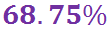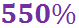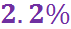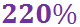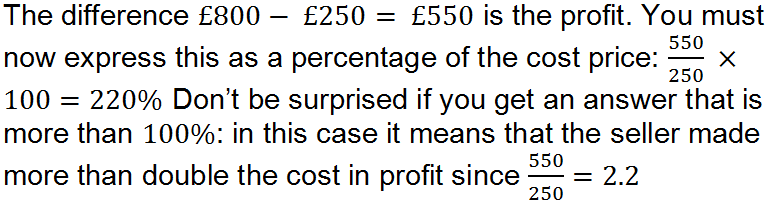Question 2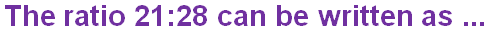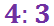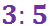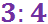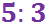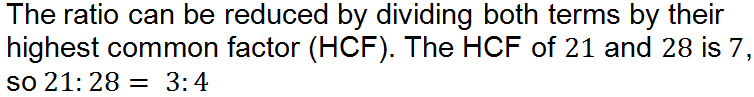Question 3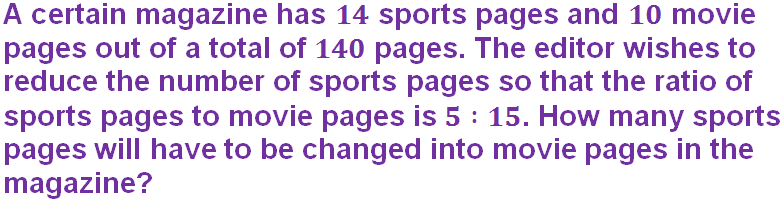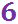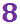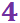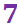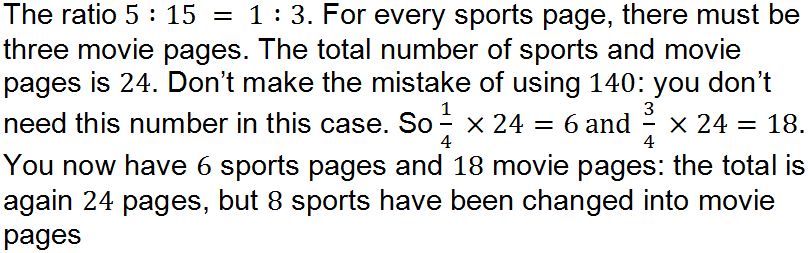Question 4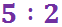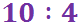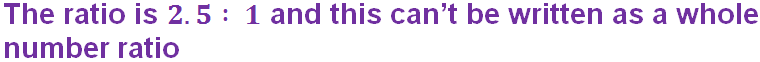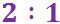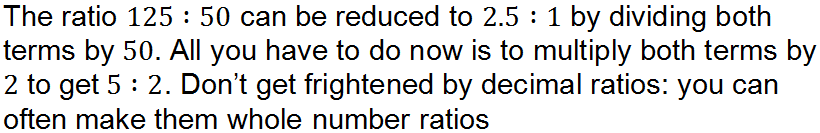Question 5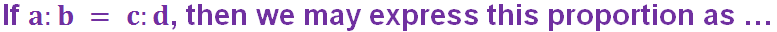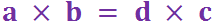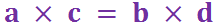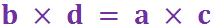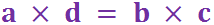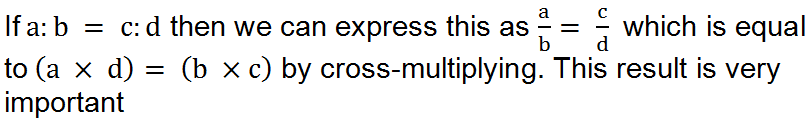Question 6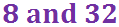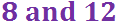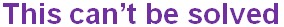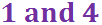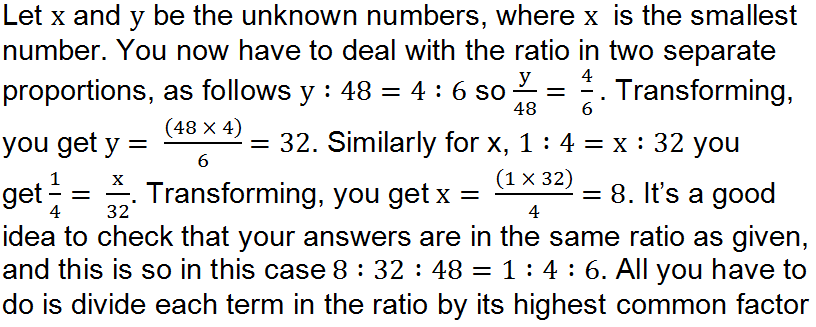Question 7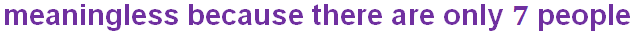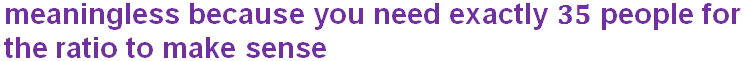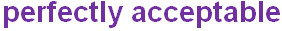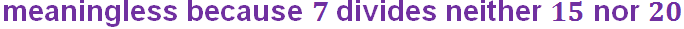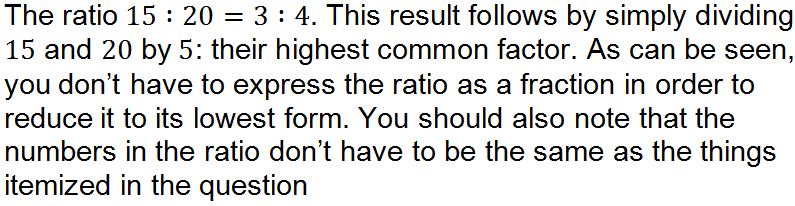Question 8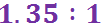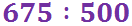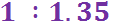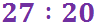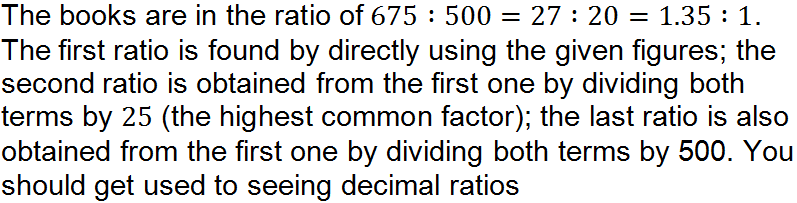Question 9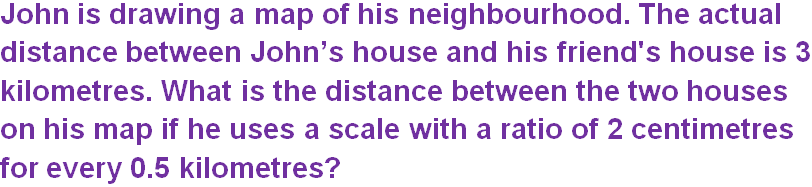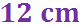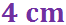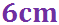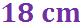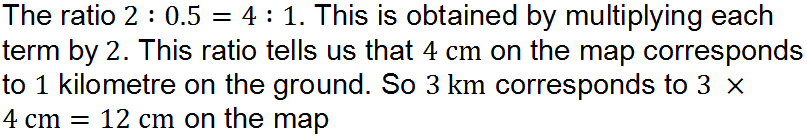Question 10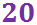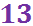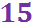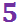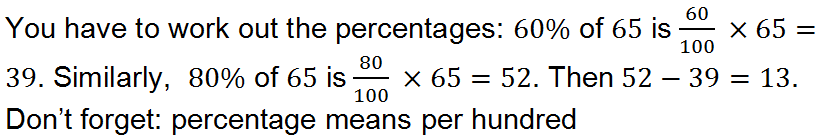Author:  Frank Evans